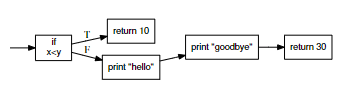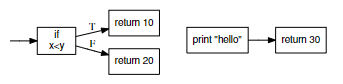# 编译器笔记--代码优化（高级优化）

## 优化视角

• 检查程序的整体算法复杂性：改进程序的算法可能比低级优化产生更大的好处。
• 衡量程序的性能。使用一些标准分析工具来准确测量您的程序大部分时间花费在哪里，然后通过重写或启用适当的编译器优化来改进该代码。

## 高级优化

### 常量折叠

``````const int seconds_per_minute=60;
const int minutes_per_hour=60;
const int hours_per_day=24;
int seconds_per_day = seconds_per_minute * minutes_per_hour * hours_per_day;``````

``````struct expr * expr_fold( struct expr *e )
{
expr_fold( e->left )
expr_fold( e->right )
if( e->left and e->right are both constants ) {
f = expr_create( EXPR_CONSTANT );
f->value = e->operator applied to e->left->value and e->right->value
expr_delete(e->left);
expr_delete(e->right);
return f;
} else {
return e;
}
}``````

### 循环展开

``````for(i=0;i<400;i++) {
a[i] = i*2 + 10;
}``````

``````for(i=0;i<400;i+=4) {
a[i] = i*2 + 10;
a[i+1] = (i+1)*2 + 10;
a[i+2] = (i+2)*2 + 10;
a[i+3] = (i+3)*2 + 10;
}

// 或者

for(i=0;i<400;i++) {
a[i] = i*2 + 10;
i++;
a[i] = i*2 + 10;
i++;
a[i] = i*2 + 10;
i++;
a[i] = i*2 + 10;
}``````

``````a = 0 + 10;
a = 2 + 10;
a = 4 + 10;
. . .``````

### 代码提升

``````for(i=0;i<400;i++) {
a[x*y] += i;
}

// 提升为

t = x*y;
for(i=0;i<400;i++) {
a[t] += i;
}``````

### 函数内联

``````int quadratic( int a, int b, int x ) {
return a*x*x + b*x + 30;
}
for(i=0;i<1000;i++) {
}``````

``````for(i=0;i<1000;i++) {
y = 10*(i*2)*(i*2) + 20*(i*2) + 30;
}``````

``````for(i=0;i<1000;i++) {
y = 40*i*i + 40*i + 30;
}``````

### 死代码检测和消除

``````if( x<y ) {
return 10;
} else {
print "hello";
}
print "goodbye";
return 30;``````return 语句会导致函数立即终止，并且（从控制流图的角度来看）是执行路径的结束。在这里，if 语句的 true 分支立即返回，而 false 分支则继续执行下一个语句。对于 x 和 y 的某些值，有可能到达每个语句。然而，如果我们做一些小小的改变，就像这样：

``````if( x<y ) {
return 10;
} else {
return 20;
}
print "goodbye";
return 30;``````